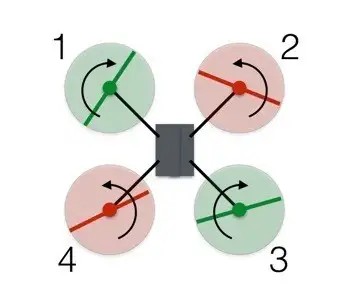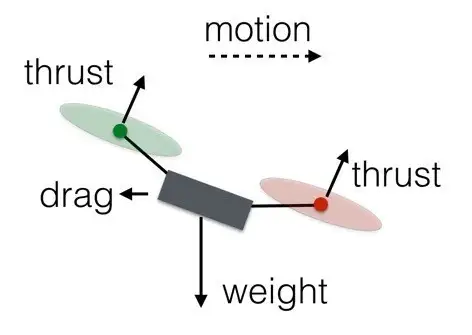Today's drones can do three things: hover, climb and lower. When hovering, the thrust generated by the drone's four rotors is equal to the downward force of gravity. It's very easy to understand. So how to achieve the climb? Increase the thrust of the four rotors to create an upward force greater than gravity. After the action is completed, the thrust of the UAV can be relatively reduced, but in order to make it continue to fly upwards, it must still be ensured that the upward force is greater than the downward force. The requirement for lowering the drone is the opposite: the thrust speed of the rotor needs to be reduced, so that the resultant force is downward. the

Rotate
How to make a drone that is flying north turn around and fly south? What is the principle of motion of the rotor at this time?As shown in the picture, the red rotors rotate counterclockwise and the green rotors rotate clockwise. When the two sets of rotors rotate in opposite directions, the total power of the drone is zero. Angular dynamic values are much like linear dynamic values and can be calculated by multiplying angular velocity by moment of inertia. It can be said that the angular power depends on the speed at which the rotor spins. the
Assuming that the red rotor has a positive angular momentum, and the green rotor has a negative angular momentum, the value of each rotor is divided into +2, +2, -2, -2, then all the forces at this time plus up to zero. The drone can hover. the
To turn the drone to the right, the angular velocity of rotor 1 needs to be reduced. However, while the lack of thrust from rotor 1 allows the drone to change direction, at the same time the upward force is not equal to the downward force of gravity, so the drone will descend. So, how do you make the drone maintain the same altitude when changing direction? the
While decreasing the rotation speed of rotors 1 and 3, increase the rotation speed of rotors 2 and 4. At this time, the angular power of the rotor is still not zero, so the drone can rotate. While the total force is still equal to gravity, the drone can remain at the same height. Since the rotor angles rotating in the same direction are diagonal, the drone can still maintain balance. the

Forward flight and sideways flight
What is the difference between the forward and backward movement principles of the drone? Actually no, because drones are symmetrical. The same applies to lateral movement. A quad drone is like a car with each side as a front, so how to move forward also explains how to move backward or to the sides. the
So how to do it?Increase the spin rate of rotors 3 and 4 and decrease the speed of rotors 1 and 2. At this point, the total thrust is equal to the weight, so the drone can maintain a constant altitude. Also, since one rotor at the rear spins counterclockwise and the other spins clockwise, the added rotational force will still be zero, and the same will be true for the rotor at the front. So overall the direction of the drone will not change. However, the added force on the rear rotors of the drone will tip it forward, so the thrust on all rotors should be increased slightly to produce a net thrust, a component of which can be used to balance the weight and force of forward motion.
the

Learn to use computer systems

Every action of operating the UAV is done by changing the speed of one or more rotors. In order to control all the rotors with one controller, some kind of computer control system must be used. In addition, the drone's accelerometers and gyroscopes allow for fine-tuning of each rotor, further improving the ease and stability of flight. So flying a drone is fairly easy if you let the computer system do all the work. the
Isn't it interesting to understand how drones fly?